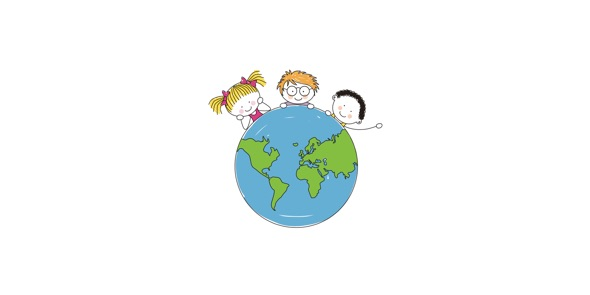# MS. Zaruba's 7th Grade Measurement Mini-quiz

Approved & Edited by ProProfs Editorial Team
At ProProfs Quizzes, our dedicated in-house team of experts takes pride in their work. With a sharp eye for detail, they meticulously review each quiz. This ensures that every quiz, taken by over 100 million users, meets our standards of accuracy, clarity, and engagement.
| Written by Rzaruba
R
Rzaruba
Community Contributor
Quizzes Created: 2 | Total Attempts: 300
Questions: 5 | Attempts: 75SettingsStandard measurement quiz for 7th graders

• 1.

### The faucet in your 70-gallon bathtub is not working. You will use a 2 quart pitcher to fill the tub halfway. How many pitchers of water will you need?

• A.

35

• B.

70

• C.

140

• D.

280

B. 70
Explanation
To fill the 70-gallon bathtub halfway using a 2-quart pitcher, you need to calculate how many times the pitcher can be filled in 70 gallons. Since there are 4 quarts in a gallon, the bathtub contains 70 x 4 = 280 quarts. And since each pitcher can hold 2 quarts, you will need 280 / 2 = 140 pitchers of water to fill the tub halfway.

Rate this question:

• 2.

### A cell phone weighs about 3.5 ounces. A portable DVD player weighs about 2 pounds. About how many cell phones equal one DVD player?

• A.

0.2

• B.

4

• C.

8

• D.

32

C. 8
Explanation
To find out how many cell phones equal one DVD player, we need to convert the weight of the DVD player to ounces. Since there are 16 ounces in a pound, the DVD player weighs 32 ounces. Now, we can divide the weight of the DVD player (32 ounces) by the weight of one cell phone (3.5 ounces) to find the number of cell phones equal to one DVD player. The result is 9.14, which can be rounded down to 8. Therefore, 8 cell phones equal one DVD player.

Rate this question:

• 3.

### How many more fluid ounces are in 2  3/4 pints than 4  1/4 cups?

• A.

10

• B.

12

• C.

16

• D.

8

A. 10
Explanation
To find the difference in fluid ounces between 2 3/4 pints and 4 1/4 cups, we need to convert the measurements to the same unit. There are 16 fluid ounces in 1 pint, so 2 3/4 pints is equal to 44 fluid ounces. There are 8 fluid ounces in 1 cup, so 4 1/4 cups is equal to 34 fluid ounces. The difference between 44 and 34 is 10, so there are 10 more fluid ounces in 2 3/4 pints than in 4 1/4 cups. Therefore, the answer is 10.

Rate this question:

• 4.

### How many inches are in 2 yards?

• A.

36

• B.

40

• C.

60

• D.

72

D. 72
Explanation
There are 36 inches in 1 yard, so to find the number of inches in 2 yards, we multiply 36 by 2. Therefore, there are 72 inches in 2 yards.

Rate this question:

• 5.

### Which is greatest in length?

• A.

1/4 of a mile

• B.

1000 yards

• C.

1200 feet

• D.

9600 inchesBack to top